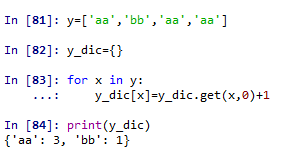### python语言的高级数据结构

• 单个元素的数值类型的转换
• 字符串str转数值型int
• 数值型int转字符串型str
• 字符串
• 字符串能直接用于循环
• 字符串中统计符号频数.count()
• 字符串的符号拆开转成List
• 数组
• 1)一维数组的创建np.arange
• 2) 多维数组的创建np.array
• 3)查看数组的相关信息【.dtype .shape type() .size .item() len()】
• 4) 创建(全1，全0，等差数列linspace，重复元素的序列)
• 5）转置X.T
• 6)数组取行、列
• 数组离散取值（索引）
• ⑦数组添加新元素
• 数据框pd.DataFrame()
• ①创建数据框
• ②修改已有的数据框的行名和列名
• ③数据框取行、列
• ④按行操作和按列操作dfname.apply
• ⑤数据框合并
• ⑥取数据框内的某个具体元素
• ⑦利用pandas读取数据文件
• 集合set()
• 列表list
• 列表按照等差的方式取值
• 给列表元素排序——后缀.sort()和函数sorted()
• 列表是值不变类型（地址id）
• 删除列表的最后一个值并返回删除值.pop()
• 在列表后面添加元素.append()和.extend()
• 查看列表中某个元素出现的次数.count()
• 随机(np.random)
• 随机种子(np.random.seed)
• 产生随机数
• 普通随机数
• 特殊分布的随机数
• 对某个序列进行重新排列np.random.permutation
• 统计频数
• 利用collections模块中的Counter()函数
• 利用pandas包下面的value_counts()函数
• 利用字典的键值来统计dictname.get()

# 单个元素的数值类型的转换

## 字符串str转数值型int

``````a='3'
print('a的数值类型',type(a))
a1=int(a)
print('a1的数值类型',type(a1))
``````
• 1
• 2
• 3
• 4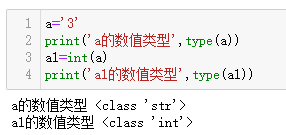## 数值型int转字符串型str

``````b=5
print('b的类型',type(b))
b1=str(b)
print('b1的类型',type(b1))
``````
• 1
• 2
• 3
• 4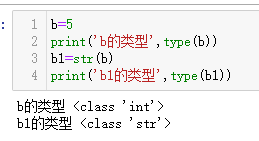# 字符串

## 字符串能直接用于循环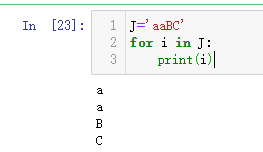## 字符串中统计符号频数.count()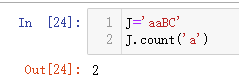## 字符串的符号拆开转成List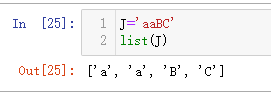# 数组

## 1)一维数组的创建np.arange

``````#任务：创建一维数组创建
import numpy as np
a=np.arange(6)
b=np.arange(-2,4,0.5)
c=np.random.randn(5)
``````
• 1
• 2
• 3
• 4
• 5## 2) 多维数组的创建np.array

• 注意这里用np.array()函数的时候，在函数括号内还必须用()或者[]把[1,2.2,3]和[2,5,8]两个括起来，否则会报错
• 一旦数组里有一个元素为浮点float形式，整个数组的元素都是浮点数
``````#任务：创建多维数组
an=np.array([[1,2.2,3],[2,5,8]])
an1=np.array(((1,2),(3,5),(7,4)))
bn=np.array(((2.3,4,6),[3,5,7]),dtype=complex)
cn=np.array([np.arange(4),np.arange(3)])
``````
• 1
• 2
• 3
• 4
• 5## 3)查看数组的相关信息【.dtype .shape type() .size .item() len()】

``````print(an.dtype) #查看数组内元素的数据形式
print(an.shape) #查看数组是几乘几的，显示数组每一维的长度
print(type(an))
``````
• 1
• 2
• 3``````.size   #显示变量中有多少个元素，若为一维数组则类似len()
``````
• 1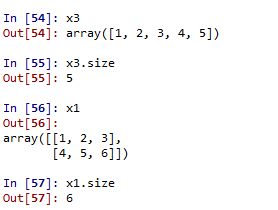``````.item()
#只能将大小为1的数组转换为Python标量，这里的大小指的就是size
``````
• 1
• 2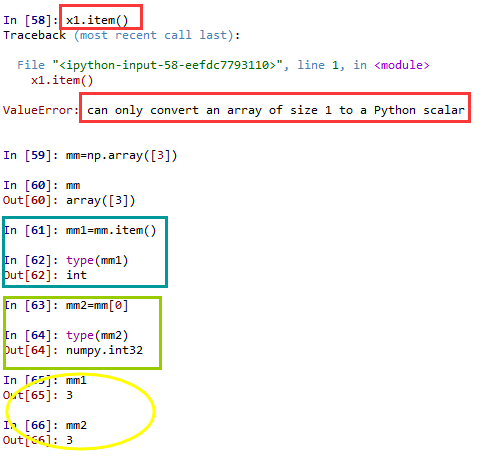``````len() #只显示数组第一维的长度
``````
• 1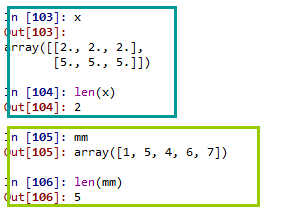## 4) 创建(全1，全0，等差数列linspace，重复元素的序列)

①全0数组np.zeros(shape，dtype，order)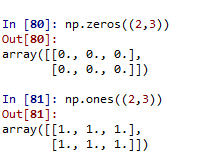②创建等差数列linspace(start,stop,num=50,endpoint=True)

``````np.linspace(2,8,10)#任务创建等差数列，默认包含终止值
``````
• 1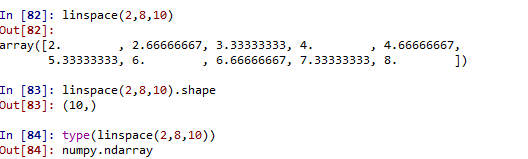③创建重复元素的序列(1维)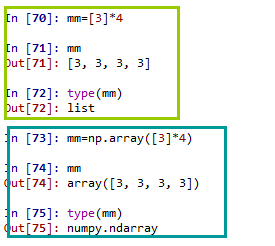④创建重复元素的二维数组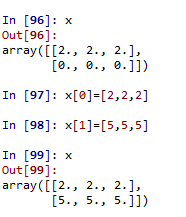## 5）转置X.T

``````x1=np.array([1,2,3,4,5,6]).reshape(2,3)
x2=x1.T
``````
• 1
• 2

## 6)数组取行、列

``````data=np.array([1,2,3,4,5,6]).reshape(3,2)
print(data)
print(data)#取行
print(data[:,0])#取列
``````
• 1
• 2
• 3
• 4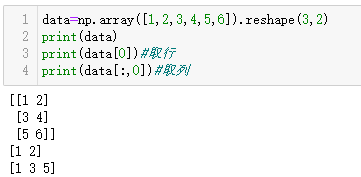## 数组离散取值（索引）

``````x=np.array([3,6,5,2,1,5])
index=[2,4]
index1=np.array([3,5])
print(x[index])#索引是列别list
print(x[index1])#索引是数组离散值
``````
• 1
• 2
• 3
• 4
• 5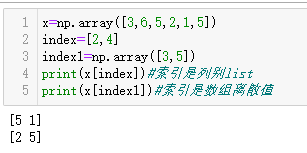## ⑦数组添加新元素

``````import numpy as np
a=np.array([1,2,3,4])
a=np.append(a,5)

import numpy as np
a=np.array([1,2,3,4])
a=np.concatenate((a,[6,7]))#注意这里输入的是元组tuple
``````
• 1
• 2
• 3
• 4
• 5
• 6
• 7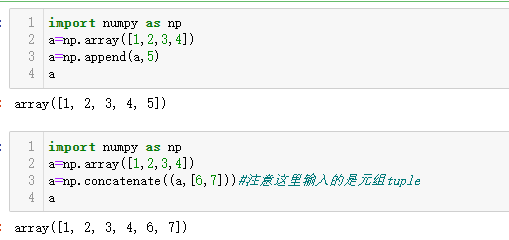# 数据框pd.DataFrame()

## ①创建数据框

``````import pandas as pd
data1 = pd.DataFrame({  'name':['wencky','stany','barbio'],
'age':[29,29,3],
'gender':['w','m','m'] } ,index=['A','B','C'] )
data1
``````
• 1
• 2
• 3
• 4
• 5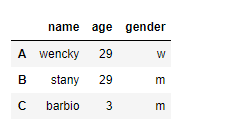``````b=np.array([1,2,3,4,5,6,6,7,8]).reshape(3,3)
data2=pd.DataFrame(  b ,columns=['l1','l2','l3'],index=['one','two','three'])
data2
``````
• 1
• 2
• 3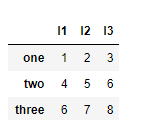## ②修改已有的数据框的行名和列名

``````dfname.index=['a','b','c']#改行名
dfname.columns=['A','B','C']#改列名
#只改某一个列的名字
df1.rename(columns={'name':'stu_name','class':'stu_class'},inplace=True)
print(df1)
#只改某一行的同理
``````
• 1
• 2
• 3
• 4
• 5
• 6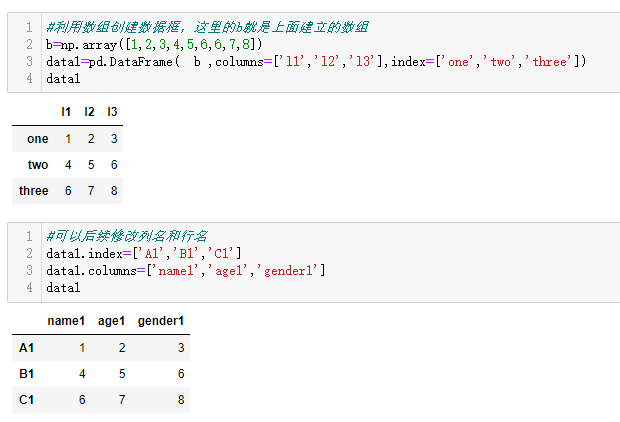## ③数据框取行、列

``````dfname[] #取第3列
dfname[15:16] #取第15行，不能少了后面的:16,不然报错
dfname.loc[index]
#这里的Index可以为[FALSE,FALSE,TRUE],也可以为[1,2,5,7]
``````
• 1
• 2
• 3
• 4

``````dfname[]  #取出索引为3的列，type(dfname[])为pandas.core.frame.dataframe
np.array(dfname[])   #转换成数组形式，是n*1维的数组
np.array(dfname[])[:,0]  #取出数组的某一列，这时n*1数组
list(np.array(dfname[])[:,0])  #这样才是list
``````
• 1
• 2
• 3
• 4
``````#python数据框取多列
dfname[['col1','col2','col5']]
``````
• 1
• 2

## ④按行操作和按列操作dfname.apply

``````def myfunc(x):
return x.max()
dfname.apply(myfunc,axis=1)#按行进行操作
#默认axis=0按列操作
``````
• 1
• 2
• 3
• 4

## ⑥取数据框内的某个具体元素

``````#第一种
df.at[i,columns]
#第二种
df.get_value(i,columns)
``````
• 1
• 2
• 3
• 4

## ⑦利用pandas读取数据文件

``````#读取csv文件

#读取xlsx文件
import pandas as pd
data3.tail(3)#显示数据的后三行
``````
• 1
• 2
• 3
• 4
• 5
• 6
• 7
• 8
• 9

# 集合set()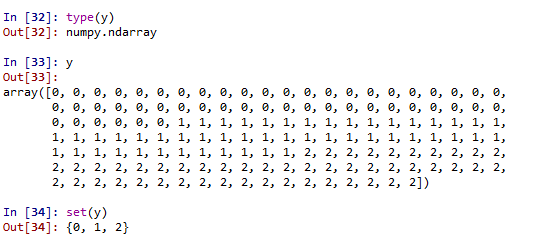# 列表list

## 列表按照等差的方式取值

• 默认情况下用切片是连续取值，即步长为1，如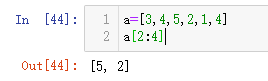• 也可以设定其他步长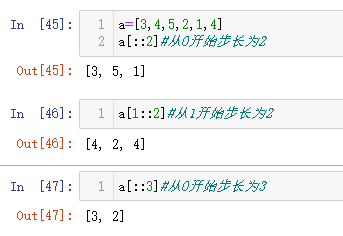## 给列表元素排序——后缀.sort()和函数sorted()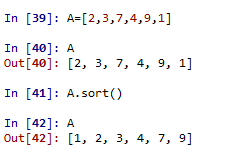sorted()函数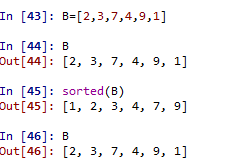sorted()运用于其他可迭代对象的示例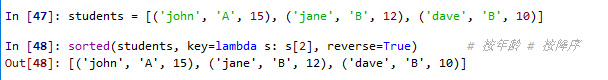## 列表是值不变类型（地址id）

``````cc=[1,2,3]
dd=cc
#可以采用这种方式改变地址
aa=list(np.array(cc))

print(id(cc))
print(id(dd))#与cc地址一致
print(id(aa))#与cc地址不一致
``````
• 1
• 2
• 3
• 4
• 5
• 6
• 7
• 8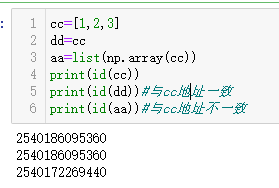## 删除列表的最后一个值并返回删除值.pop()

``````#pop不含参数默认删掉最后一个元素
a=[3,4,6,7,8]
print('删除值',a.pop())
print('删除后a是',a)
``````
• 1
• 2
• 3
• 4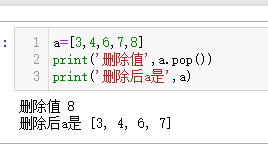``````#也可以给pop指定参数，用于删除列表中特定位置的元素
a=[3,4,6,7,8]
print('删除第3个值',a.pop(2))
print('删除后a是',a)
``````
• 1
• 2
• 3
• 4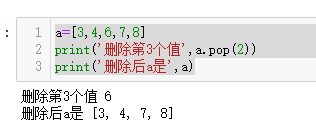## 在列表后面添加元素.append()和.extend()

• 首先.append(列表new)是把列表new当作一个整体加到原列表后面
``````a=[3,4,6,7,8]
b=[1,2,3]
a.append(b)
print('添加后a为',a)
print('b为',b)
``````
• 1
• 2
• 3
• 4
• 5
• 另一个.extend(列表new)是把列表new中的元素一个一个加到原列表后面。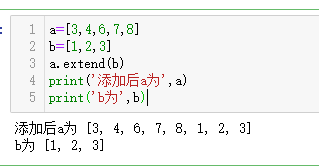## 查看列表中某个元素出现的次数.count()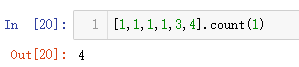# 随机(np.random)

## 随机种子(np.random.seed)

python需要对用到的每个包都设置相应的随机种子，包括
random.seed(1234) ——-这个对应于random模块
numpy.random.seed(1234) ——-这个对应于numpy模块

``````import numpy as np
#设种子
np.random.seed(123)
``````
• 1
• 2
• 3

## 产生随机数

### 普通随机数

``````# 产生0-1之间均匀分布的随机数
np.random.rand(2,3)  #2*3维的矩阵
#产生标准正态分布的随机数
np.random.randn(3,4)  #3*4维矩阵
#产生区间内[1,100)整数随机数
np.random.randint(1,100,[2,3])#2*3维矩阵
#产生[0,1)之间的随机浮点数float
np.random.random(10) #只有一维
np.random.random([2,3])#产生[0,1)之间2*3维的矩阵
``````
• 1
• 2
• 3
• 4
• 5
• 6
• 7
• 8
• 9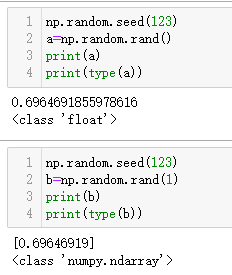### 特殊分布的随机数

``````# 正态分布mu=3,sigma=9
np.random.normal(3,9,[2,3])
#F分布，自由度为3,9
np.random.f(3,9, 1000)
``````
• 1
• 2
• 3
• 4

## 对某个序列进行重新排列np.random.permutation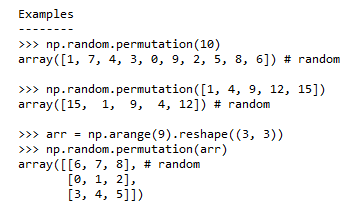# 统计频数

## 利用collections模块中的Counter()函数

Counter函数用于统计频数并以字典的形式返回，字典的键就是属性值，字典中对应的键值就是属性出现的频数。

• 统计字符串中字母的频数
`cc.most_common(3)#只显示频数最大的前三个`
`list(cc.elements())#显示所有元素，只是将相似的元素全放一起`
`cc.keys()和cc.values()类似字典用法`
``````from collections import Counter
cc=Counter('abcdeabcdabcababb')
cc
cc.most_common()#按顺序输出所有的，形式为列表，元素为二元对（‘b’,6）
cc.most_common(3)#只显示频数最大的前三个

cc.elements()   #把相似的元素放在一起
#这个返回的结果是迭代器，不是一个列表所以需要手动让它显示
print(list(cc.elements()))
#等价于
print([x for x in cc.elements()])
#或者用sorted排序也行,因为sorted()可以用于所有可迭代的对象
print(sorted(cc.elements()))

cc.keys()
cc.values()
sum(cc.values())

d = Counter('simsalabim')
cc.update(d) #这应该是字典的用法，合并字典操作
Counter('abbb')+Counter('bcc')#直接用加号合并两个频数表
Counter('abbb')&Counter('bcc')#两个字符串重复元素频数表
Counter('abbb')|Counter('bcc')#取两个频数表中元素值频数最大的组成频数表

cc.clear()#清空频数表Counter的所有内容
``````
• 1
• 2
• 3
• 4
• 5
• 6
• 7
• 8
• 9
• 10
• 11
• 12
• 13
• 14
• 15
• 16
• 17
• 18
• 19
• 20
• 21
• 22
• 23
• 24
• 25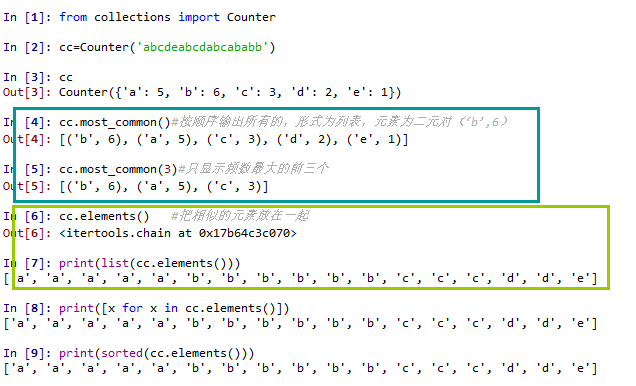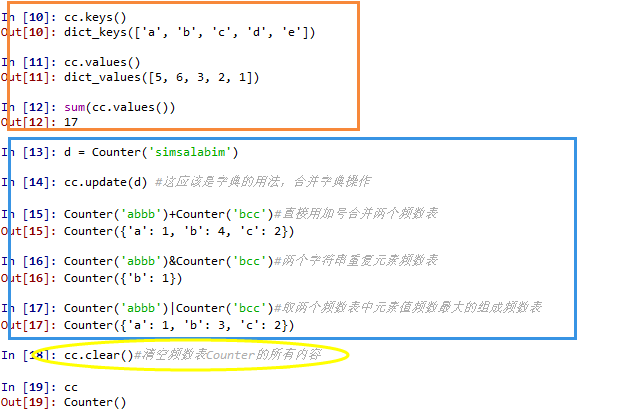• 对列表list进行Counter频数统计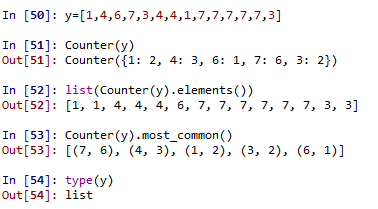• 对数组np.array进行Counter频数统计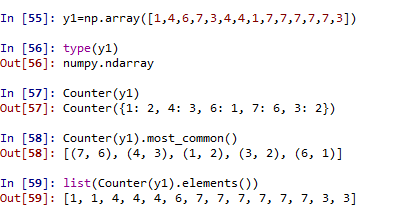• 对数据框类型pd.DataFrame进行Counter频数统计
注意：不能直接对数据框进行频数统计，需要先转化成数组形式或列表形式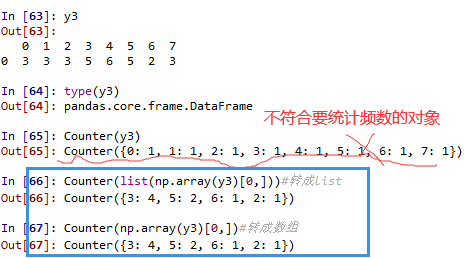## 利用pandas包下面的value_counts()函数

• 对列表list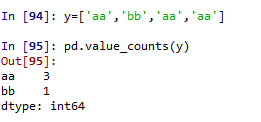• 对数组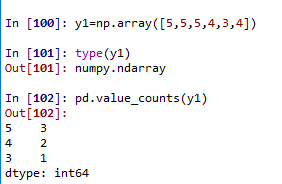## 利用字典的键值来统计dictname.get()

①对字符串②对列表